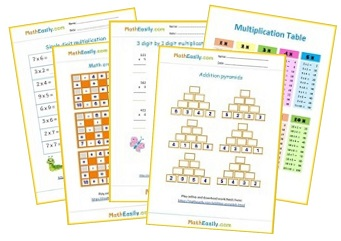﻿ 100+ Free Math Games for Grade 5 ONLINE Practice

Free Online Math Games > 5th Grade Math

# Free Math Games for Grade 5

Play online 5th Grade Math Games for free. Check results, find mistakes. Our online math games for Grade 5 help kids to practice addition, subtraction, multiplication, division and much more. These free math games for Grade 5 contain also worksheets on which kids can practice a certain skill. You can find all our 5th Grade math worksheets here.

These fifth grade math games has been carefully designed to cover sub-topics under the main topics listed below. Teachers, parents and students can use them for 5th Grade online math practice.

## Online 5th Grade Math Games

### Math picture puzzles

Play free math games for 5th graders. Interactive picture puzzles improve logic skills. Solve the picture equations.
Play now

### Fractions on a number line

Placing fractions on a number line´ games. Select the fraction that corresponds to the given point on the number line.
Play now

Play now

### 2 digit by 2 digit multiplication games

Learn how to multiply 2x2 digit numbers. Watch the tutorial, find mistakes. Play 5th grade math games online.
Play now

You can choose between 2 options: 3 digit addition games with or without regrouping. Play free online math games for grade 5.
Play now

### 3 digit subtraction games

Practice 3 digit subtraction online. Free 5th grade math games: 3 digit subtraction with or without regrouping games.
Play now

### Math Halloween puzzles

Solve Halloween math puzzles. Download math Halloween emoji puzzles in PDF. Play Hallowen math games online.
Play now

Play now

## Online Math Games for Grade 5

Play our free math learning games for 5th graders, that teach multiplication and division:

### Multiplication pyramids

Learn to multiply within 500. Play multiplication pyramid games online. Free worksheets are included.

Play now

### Multiplication flash cards

Online multiplication flash cards help kids to master multiplication. Printable flash cards included.

Play now

### Division flash cards

Practice division and play free math games for grade 5: online division flash cards 1-12.

Play now

### 2 digit by 1 digit multiplication

Learn how to multiply two by one digit numbers. Play interactive math games for 5th graders.

Play now

Play now

### 3 digit by 2 digit multiplication

Multiply three digit by 2 digit numbers. Play online games, download worksheets.

Play now

### 3 digit by 3 digit multiplication

Learn how to multiply 3x3 digit numbers. Watch the tutorial, download worksheets.

Play now

All free multiplication games →

All free division games →

### Interactive math games for 5th Grade

Interactive math games for 5th Grade are supported by math worksheets. Here are the most popular worksheets which help students to improve 5th Grade math skills. You can find all our math worksheets for Grade 5 here.## Free 5th Grade Math Games

Prefer to stay paperless? Our free online math games for Grade 5 are here for students learning virtually.

Capturing your students' interest and curiosity during the first few minutes of math class is the key to keeping them engaged for your entire lesson.

These interactive math games for 5th garders will help you with this. Our maths puzzles are fun and easy to solve.

Pin it for later!

## Free Online Math Games for 5th Grade

Learning math is about practicing. Need more exercises? Then try also these free online 4th grade math games.Free Math Worksheets